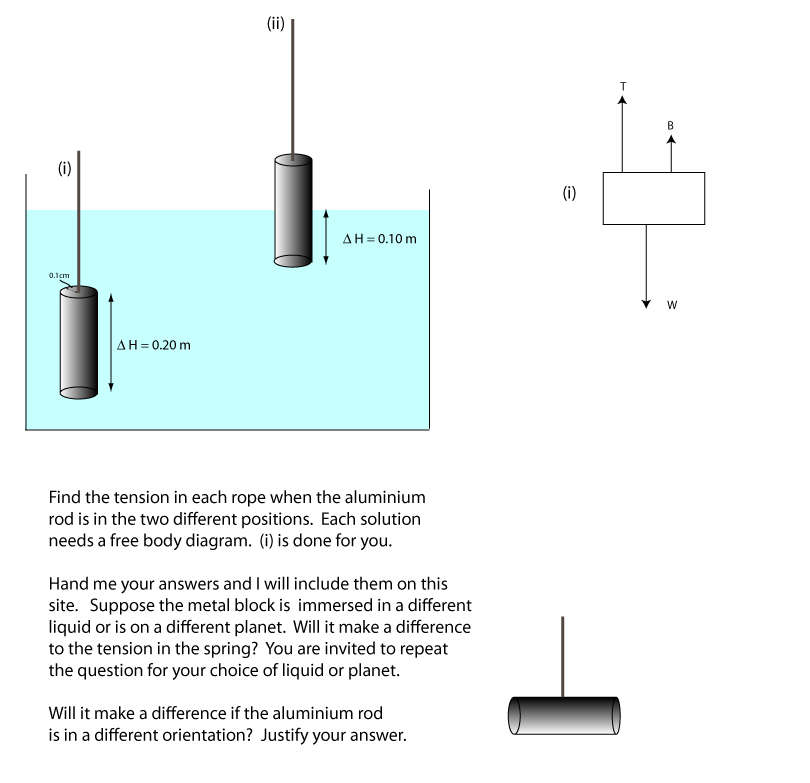Aids to Understanding

Density issues

If we have two objects of equal volume but one body is twice as heavy, then the heavier object is twice as dense. The particles that make up the body are more tightly packed. The property of density applies to liquids, solids and gases. It should be clear that volume, but not mass, changes with temperature so when we state the density of a solid we understand it is the density at room temperature. The same applies for a gas but the pressure of the gas is important. The density of a gas like air is usually quoted at room temperature and atmospheric pressure.

Conversion Factors

The density of a substance is the mass of the substance divided by its volume. It can be stated in units of
kg m-3 or
g cm-3 or
g (or kg) l-1
Converting is easy but it is also something that has to be learned.

Example

The element Osmium has the highest density of any

substance on Earth, 22550 kg m-3. State its density in units of g cm-3.
1 kg = 1000g
So 22550 kg m-3 becomes
22550000 g m-3
1 m3 = 106 cm3 so
1 m-3 = 10-6cm-3
so 22550000 g m-3
becomes 22.55 g cm-3

Example

The density of gold is 19.3 g cm-3, express this in units of kg m-3. Multiply the figure by 1000 to get the answer but make sure you know what you are doing.

Specific Gravity

On the table of common densities, water is highlighted. It is no accident water has a density of exactly 1000 kg m-3. It is defined as such. The densities are sometimes quoted with reference to this number. For example we might say Aluminium has a specific gravity of 2.7, this simply means its density is 2.7 times greater than water. It would be better if it was called specific density, but the name has stuck for historical reasons. You are invited to find out.

Density and flotation

You might already know that if a body has a specific gravity greater than 1 it will sink in water, but less than 1 it will float. This is good working knowledge but it does not explain the phenomenon. However, after more work on this webpage, we will soon know why.

Resolving Force vectors

We resolved resulant forces in to their horizontal and vertical components in 1.1. It might be useful to revisit this. If the force is acting at an angle to the surface then for the purposes of pressure calculations we must work out the vector normal to the surface. In the diagram below if the force is 60 N and the angle is 30o then the component Of force at right angles to the surface is 60sin30 = 30 N. (the component parallel to the slope that we found a use for in a previous section is simply 30 cos 30 = 15√ 3 N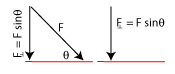Pressure in liquids

In this course the liquids we study are liquids at rest. In a liquid at rest the unbalanced force acting on all molecules (or any cross section of the liquid), is zero. It follows that the pressure at a point (any point) in a liquid is acting in all directions. The diagram below helps to illustrate this important point.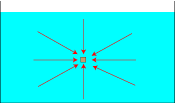The red vectors can represent either force or pressure vectors. Remember P = F/A so if F is a vector so is P.

The Buoyancy force

The buoyancy force is all around us. For example the lift experienced by a helium filled balloon is due to the natural buoyancy of the air. Because the upper air pressure on the balloon is less than the lower air pressure, the balloon experiences a lift. If the buoyancy force is greater than the weight of the ballon, the balloon rises (floats). The same point applies to floating bodies in water. Try to show for yourself that if the density of the floating object is less than the density of the fluid surrounding it, then the body floats, otherwise it sinks.

For a given fluid and gravitational field strength, the buoyancy force on a body depends only on the dimensions of the body. If the body is fully submerged then the buoyancy force acting on it does not change with depth. Although the deeper the body becomes the greater the pressure it experiences and becomes more squashed. For example divers cannot dive to any more than 300m. The world record for a scuba dive is 330 m.

...a misconception...

You may have heard that air inside bodies makes the body float and the more air you pump into it the more likely it is that this air makes it float. This is not quite right. If air is pumped into a body and the body is allowed to expand then its volume increases with no appreciable gain in mass. Therefore the buoyancy due to its environment increases.

Archimedes Principle

This is for interest only.
The following problem yeilds a new way to think about the buoyancy force.

Ex;

Suppose a 1 m3 solid cube is completely submerged in water. i) What is the volume of water displaced by this cube?
ii) Calculate the buoyancy force on the cube.
iii) Calculate the weight of water displaced by this cube.
i) The volume of water displaced is equal to the volume of the cube, 1 m3.
ii) The buoyancy force equals
F = DPA = rgh1 - (h2)A = 1000x9.8x1x12 = 9800 N.
iii) Weight of water displaced by cube = mwaterg = rVg
= 1000x9.8x13 = 9800 N
iv) The values are the same.
This can be interpreted as follows. The buoyancy force on a body is equal to the weight of the water displaced by the body. This is known as Archimedes Principle Archimedes principle is useful when we are studying irregularly shaped bodies. All we need to know is the volume of the body and working out the bouyancy force acting on it is a simple matter. In our course though we will always be given shapes whose volumes are easilly calculated so you will be expected to use the principles discussed in the main body of this web page.

Example:

The shape in the diagram is weighed by a Newton balance and has a weight of exactly 10 N. Suppose you were asked to estimate what the reading on the Newton Balance would be if it was immersed in;
i) Water
ii)Olive oil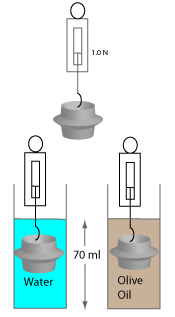Outline the steps you would take and suggest a possible answer. Look carefully at all values given and also suggest what the metal might be. Any answer you give can be posted on this site so others can discuss it. Any modifications or additions are welcome and acknowledgement will be given.

Suggestion

Using first principles, estimate the buoyancy force on a Helium filled balloon and verify using Archimedes Principle.

The Buoyancy Force and Notation

It is all very well and convenient to label the Buoyancy force on a body as B, but alwways keep in mind that the force on the body is due to the surrounding fluid and can be more formally stated (and this can make things clearer) as B = FBF ie the buoyancy force on a body is the force on the Body due to the Fluid.

## 1) Density

A tree can be much larger than a coin but a coin sinks and a tree floats. But if we cut from the tree a shape equal in dimensions to the coin, then we would notice the coin was heavier. We need a physics (quantifiable) way to think about this phenomenon and we use the concept of density. The density of a body is the mass of the body (or liquid or gas) divided by the volume it occupies. It should be fairly clear that the larger the body's mass and the smaller the body's volume then the greater its density. Density is a useful quantity in physics because it allows us to make predictions about floating bodies and whether or not a body is pure. For example, concepts like density helped Archimedes determine if his King's crown was made of pure gold. (it wasn't it turned out) In general smaller objects that feel heavy are more dense than larger bodies that are easier to lift.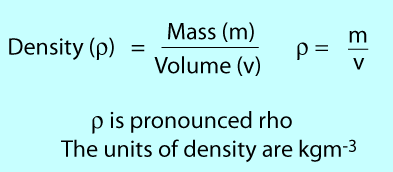## 2) Density Calculations

A density calculation always involves using mass/volume but it usually involves some sort of problem solving exercise. For example suppose an airline allows a maximum of 25 kg per suitcase. If the suitcase has dimensions 1m x 1.25m x 0.5m then find out if the three coloured blocks (densities shown) in the case together exceed the mass limit. Study the solution below and make sure you understand it.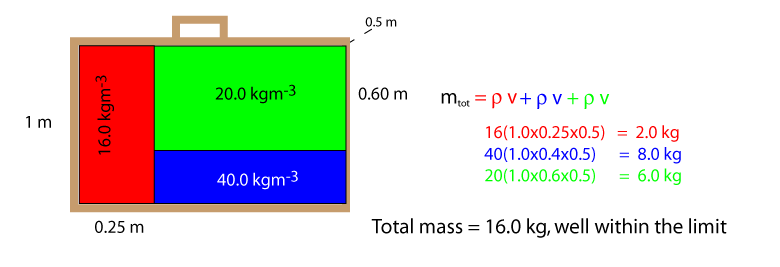## 3) The Density of air

The density of air can be found using the apparatus shown below. Study it carefully and make sure you understand the conversion factors. It is important to be able to convert from grams per cubic centimeters to kilograms per cubic meters (or the reverse) without too much fuss. Also look closely at the diagram and try to find limitations of this apparatus. For example suppose the pump is unable to completely evacuate the flask, will this give as a value of density for air that is too large or small? Or suppose the volume of the flask is slighlty less than 1.5 ml, how will this affect the calculated density. Finally what assumptions have we made about the temperature and pressure of the flask. The cotton wool is there for safety, can you think why?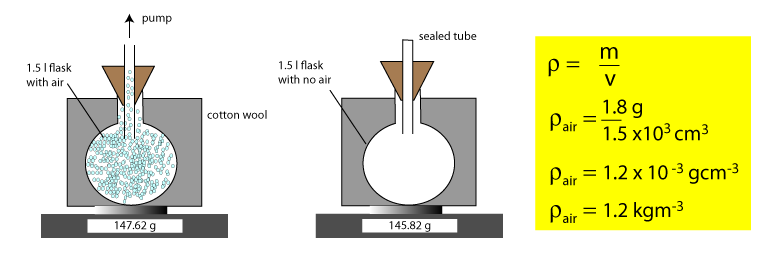## 4) Densities of Common substances.

Knowing the value of the density of one substance is of limited use. If we know the densities of a large group of solids, liquids and gases then we can look for patterns. The patterns we find here provide us with information on the arrangement of the atoms and molecules in the three states of matter. These values are for standard temperature and pressure.
Solid, liquid or gas Density (kg m-3) Density (g cm-3) Solid, liquid or gas
Cork 250.0 0.250 Solid
Olive oil 920.0 0.92 Liquid
Water 1000.0 1.0 Liquid
Air 1.29 0.00129 Gas
Carbon Dioxide 1.98 0.00198 Gas
Aluminium 2700.0 2.7 Solid
Copper 8960.0 8.96 Solid
Perspex 1190.0 1.19 Solid
Nitrogen 1.25 0.00125 Gas
Gold 19300.0 19.3 Solid

If you look at the relative densities of gases and solids then you will see that, at standard temperature and pressure, 1 kg of gas occupies round about 1000 more volume than 1 kg of a solid. This is means the average spacing of of particles in the gaseous state is ten times greater than when in the solid state. The diagram below illustrates this.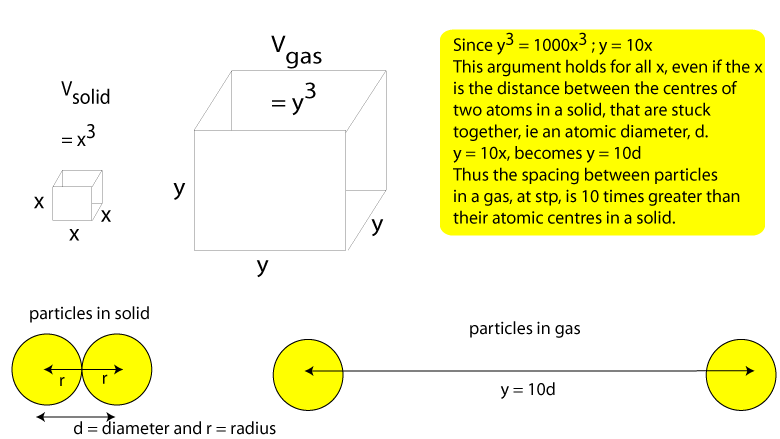## 5) Pressure

Suppose a 2000 kg elephant is led across a soft surface by 60 kg person. Which body will damage the soft surface most? There is no obvious answer to this because it depends not only on the mass of each body but the area in contact with the surface. It is fair to suggest see that a person wearing stilletto heels will probably damage the floor more than a large flat footed elephant. We need a physics (quantifiable) way to think about this phenomenon and we use the concept of pressure. It should be clear if the pressing force becomes larger and the area it is acting on becomes smaller, then the pressure will increase.

## 6) The Pascal

The Pascal is the unit of pressure and was named after Blaise Pascal the Freanch scientist. Pascal contributed much to our understanding of pressure variations in fluids. It is important to note that the force that is used in our calculations is the magnitude of the force that is at right angles (normal or perpendicular) to the surface it is acting on. If not we must resolve our force vector and use the vector perpendicular to the surface. F indicates we are using the force perpendicular to the surface.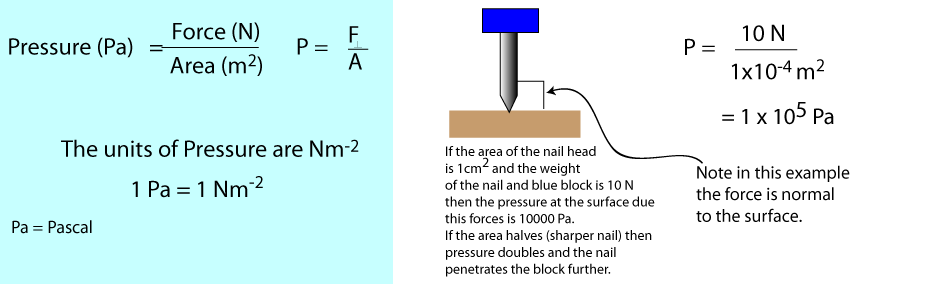## 7) Pressure Calculations

In this example you are asked to work out the pressure exerted by the block of copper in the two situations. The answers are given but you ought to work them out yourself. Notice that pressure can also be worked out using p = rgh, whenever you see another expression you should verify the units of rgh are consistent with units of pressure ie Nm-2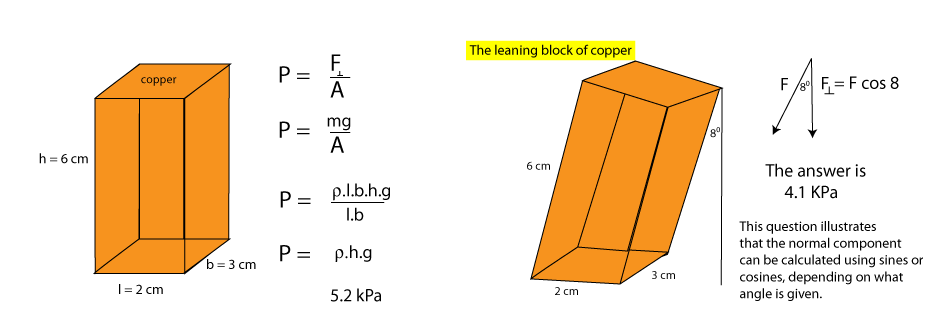## 8) Pressure in Fluids

An understanding of the characteristics of pressure in liquids enables to explain phenomena of flotation and buoyancy. If we consider a liquid at rest and ask what the pressure is at some point in the liquid, then we might say it would depend on; i) the point below the surface where we measure ii) the density of the liquid and the gravitational field the liquid is in. These are perfectly good suggestions and merit investiagation. The results are shown below. It is an important skill to be able to plan and carry out experiments. Think about what you would need and try to plan and carry these activities out in the lab.
Independent Variable Dependent Variable Controlled variables Result in Graphical form
Depth Pressure Density and Gravitational field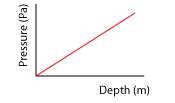Density Pressure Depth and Gravitational field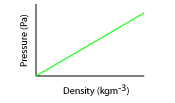Gravitational field Pressure Depth and Density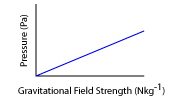The results of the experiments can be summarised by one simple expression p = rgh. When we use standard units for our measurements the constant of proportionality (gradient) for each graph above can be taken as 1.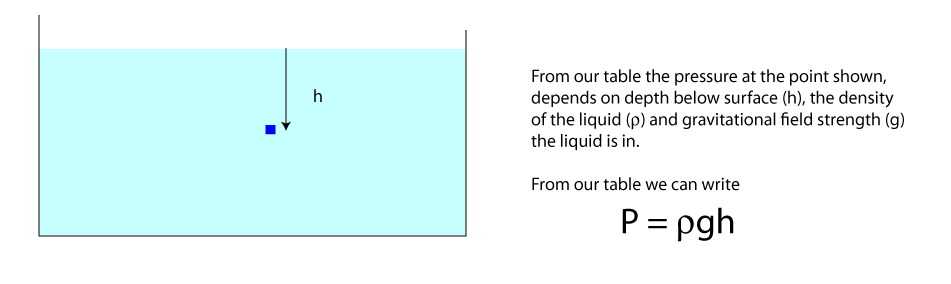## 9) Pressure in Fluids problems

Suppose a cube of alumuminium of sides 3.5 m is lowered into a fluid as shown. Calculate the pressure on the upper and lower faces on the cube and find their difference.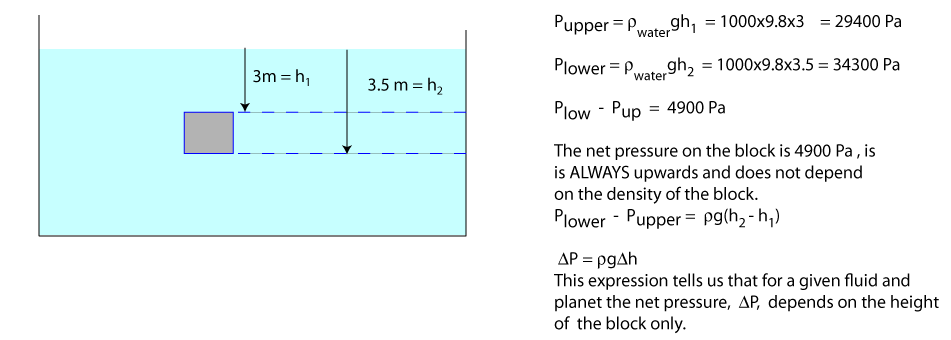## 10) The Buoyancy Force

To help explain the buoyancy force we return to the previous question and ask the following. If the net upwards pressure on the aluminium block is 4900 Pa, calculate the net upwards force. This is simply F = DP.A = 4900X3.52 = 60.0 kN. The net upwards force is called the Buoyancy force and is usually denoted B. The explanation of the buouyancy force on a fully submerged body comes up in examinations reguralarly and it is a classic 4 point question worth 2 marks (1/2 + 1/2 + 1/2 + 1/2) for each point. The four row table below indicates what these four points are.

## Explaining the bouyancy force on a fully submerged body

Point Number Principle or Point Graphical Illustration
1. The Pressure in a liquid is proprtional to depth2. The pressure on the lower surface of a body is necessarilly greater than the pressure on the upper surface.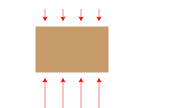3. The differences in pressure leads to a net pressure, DP, that is directed upwards4. Since F = DP.A, where A is area of the lower surface a net upwards pressure results in a net upwards force. This force is called the buoyancy force.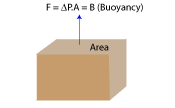Example: Suppose an aluminium rod is raised in water by a rope attached to a crane. Your job is to find the tension in the rope when the rod is i) Fully submerged, ii) Half submerged. All the information you need to solve this problem is on this webpage.